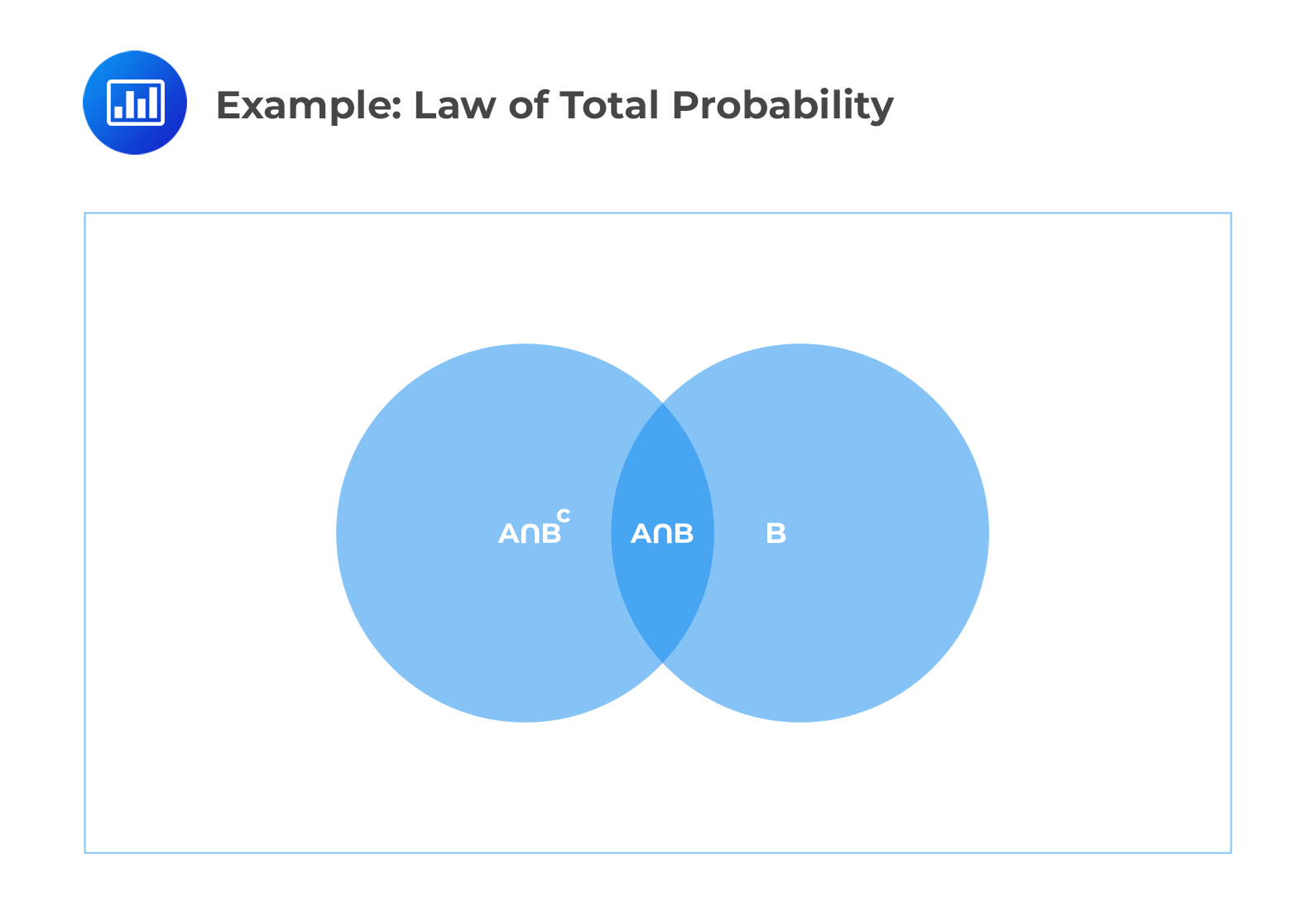Limited Time Offer: Save 10% on all 2022 Premium Study Packages with promo code: BLOG10# State Bayes Theorem and use it to calculate conditional probabilities

## Bayes Theorem

Before we move on to Bayes Theorem, we need to learn about the law of total probability.

### The Law of Total Probability

The law of total probability states that if E is an event, and $$A_1, A_2, \cdots A_n$$ are the partition of the sample space, then

$$P(E)=P(A_1 \cap E)+P(A_2 \cap E)+\cdots P(A_n \cap E)$$

We can use the law intuitively by recalling that:

$$A=(A\cap B)\cup (A\cap B^c)$$

Consider the following Venn Diagram:Note that  $$B^c$$ (also be written as $$B’$$) is the complement of an event B.

Therefore,

$$P(A)=P(A\cap B)+P(A\cap B^c)$$

Recall the conditional probability that:

$$P(A|B)=\frac{P(A\cap B)}{P(B)}$$

So that:

$$P(A\cap B)=P(B)\bullet P(A|B)$$

Similarly,

$$P(A\cap B^c)=P(B^c)\bullet P(A|B^c)$$

Thus,

$$P\left( A \right) =P\left( A|B \right) \bullet P\left( B \right) +P\left(A|B^c \right)\bullet \left( P\left( B^c \right) \right)$$

At this point, we can write the expression for $$P(B|A)$$ as:

$$P(B|A)=\frac{P(A\cap B)}{P(A)}=\frac{P(A)\bullet P(A|B)}{P\left( A|B \right) \bullet P\left( B \right) +P\left(A|B^c \right)\bullet \left( P\left( B^c \right) \right)}$$

The last expression is referred to as Bayes’ Theorem.

Similarly,

$$P(B^c |A)=\frac{P(A\cap B^c)}{P(A)}=\frac{P(A)\bullet P(A|B^c)}{P\left( A|B \right) \bullet P\left( B \right) +P\left(A|B^c \right)\bullet \left( 1-P\left( B \right) \right)}$$

### Definition: Bayes’ Theorem

If $$E$$ is an event and $$A_1 , A_2 ,…, A_3$$ are the partition of a sample space, then:

\begin{align} P(A_i |E)&=\frac{A_i \cap E}{P(E)}\\ &=\frac{P(A_i)\bullet P(E|A_i)}{P(A_1)\bullet P(E|A_1)+P(A_2)\bullet P(E|A_2)+\cdots +P(A_n)\bullet P(E|A_n)} \end{align}

Consider the following examples:

#### Example: Law of Total Probability

In a given population of students, 25% play baseball, and 30% play basketball. Also, the probability of a student playing baseball given that they also play basketball is 10%.

Calculate the probability that a student plays baseball given that they do not play basketball.

Solution

Let event $$A$$ be “plays baseball” and event $$B$$ be “plays basketball.” Using the law of total probability, we have:

\begin{align} P\left( A \right) & =P\left( A|B \right) \bullet P\left( B \right) +P\left(A|B^c\right)\bullet \left( 1-P\left( B \right) \right) \\ 0.25 &= 0.10 \bullet 0.30 + x \left(1-0.30 \right) \\ 0.22 &= x \left(0.70 \right) \\ x &= \frac{0.22}{0.70} = 0.314 \ or \ 31.4\% \end{align}

#### Example: Bayes’ Theorem

An insurance company deals with three insurance policies: 40% of life insurance, 25% of car insurance, and 35% of health insurance. The probability that a life insurance policyholder will file a claim in a given year is 0.50. The probability that a car insurance policyholder will file a claim in a given year is 0.20. Lastly, the probability that a health insurance policyholder will file a claim in a given year is 0.10.

At the course of this year, a policyholder files a claim.

Calculate the probability that the claim comes from the car insurance policyholder.

Solution

Let:

$$L$$ = proportion of life insurance policyholders.

$$R$$ = proportion of car insurance policyholders.

$$H$$ = proportion of health insurance policyholders.

$$C$$ = event that a claim is made.

From the information given in the question, we have:

$$P(L) = 0.40,\ P(R) = 0.25,\ P(H) = 0.35$$

Also, we have:

$$P(C|L) = 0.50,\ P(C|R) = 0.20,\ P(C|H) = 0.10$$

We need, $$P(R|C)$$. Using the Bayes’ theorem, we know that:

\begin{align}P(R|C)&=\frac{P(R)\bullet P(C|R)} {P(R)\bullet P(C|R)+P(L)\bullet P(C|L)+P(H)\bullet P(C|H)}\\ &=\frac{0.25\times 0.20}{0.25\times 0.20+0.40\times 0.50 +0.35\times 0.10}\\ &=0.17543 \approx 17.54\%\end{align}

Learning Outcome

Topic 1.g: General Probability – State Bayes Theorem and use it to calculate conditional probabilities.

Shop CFA® Exam Prep

Offered by AnalystPrepLevel I
Level II
Level III
All Three Levels
Featured Shop FRM® Exam PrepFRM Part I
FRM Part II
FRM Part I & Part II
Learn with Us

Subscribe to our newsletter and keep up with the latest and greatest tips for success
Shop Actuarial Exams PrepExam P (Probability)
Exam FM (Financial Mathematics)
Exams P & FM
Shop GMAT® Exam PrepComplete CourseDaniel Glyn
2021-03-24
I have finished my FRM1 thanks to AnalystPrep. And now using AnalystPrep for my FRM2 preparation. Professor Forjan is brilliant. He gives such good explanations and analogies. And more than anything makes learning fun. A big thank you to Analystprep and Professor Forjan. 5 stars all the way!michael walshe
2021-03-18
Professor James' videos are excellent for understanding the underlying theories behind financial engineering / financial analysis. The AnalystPrep videos were better than any of the others that I searched through on YouTube for providing a clear explanation of some concepts, such as Portfolio theory, CAPM, and Arbitrage Pricing theory. Watching these cleared up many of the unclarities I had in my head. Highly recommended.Nyka Smith
2021-02-18
Every concept is very well explained by Nilay Arun. kudos to you man!2021-02-13